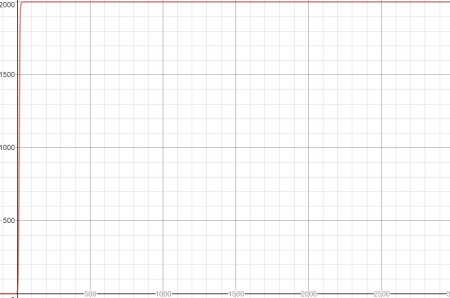# In 1774, Captain James Cook left 10 rabbits on a small Pacific island. The rabbit population is...

## Question:

In 1774, Captain James Cook left 10 rabbits on a small Pacific island. The rabbit population is approximated by {eq}P(t)= \frac{2000}{1 + e^{5.3 - 0.4t}} {/eq} with t measured in years since 1774. Use a calculator or computer to answer the following questions.

a) Graph P. Does the population level off? If it does, at what population?

b) Estimate when the population of rabbits grew most rapidly and fid the population.

c) Find the inflection point on the graph and explain its significance.

## Analyzing a Graph:

In this question, we will be analyzing the given equation by plotting its graph. It is possible to analyze a curve both algebraically and graphically. We do so over here by analyzing the derivative and the plot of the given function.

a)As is visible on the graph above, the population will level off at 2000 rabbits.

b)

We want to find the point when the rate of growth is...

Become a Study.com member to unlock this answer! Create your account